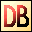DelphiBasicsWhileKeyword Repeat statements whilst a continuation condition is met
 While Expression do Statement;
Description
The While keyword starts a control loop that is executed as long as the Expression is satisfied (returns True).

The loop is not executed at all if the expression is false at the start.

You need Begin or End markers if multiple statements are required in the loop.

It is used when it is important that the statements are at only executed when necessary.
Related commands
 Begin Keyword that starts a statement block Boolean Allows just True and False values Do Defines the start of some controlled action End Keyword that terminates statement blocks For Starts a loop that executes a finite number of times Repeat Repeat statements until a ternmination condition is met Until Ends a Repeat control loop
Author links

Buy Website Traffic at
Buywebsitetrafficexperts.com

Buy Proxies at
Buyproxies.ioDownload this web site as a Windows program.

 Example code : Display integer squares until we reach 100 var   num, sqrNum : Integer; begin   num := 1;   sqrNum := num * num;   // Display squares of integers until we reach 100 in value   While sqrNum <= 100 do   begin    // Show the square of num     ShowMessage(IntToStr(num)+' squared = '+IntToStr(sqrNum));     // Increment the number     Inc(num);     // Square the number     sqrNum := num * num;   end; end; Show full unit code 1 squared = 1   2 squared = 4   3 squared = 9   4 squared = 16   5 squared = 25   6 squared = 36   7 squared = 49   8 squared = 64   9 squared = 81   10 squared = 100
 Delphi Programming © Neil Moffatt 2002 - 2018. All rights reserved.  |  Home Page